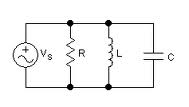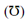## Parallel RLC Circuit Assignment Help

Assignment Help: >> Resistive - Inductive and Capacitive Circuits - Parallel RLC Circuit

Parallel RLC Circuit:

Now we connect passive elements R, L, C in parallel along with one current source.Figure: Parallel RLC Circuit

As the circuit is parallel the voltage v across all of the elements remains same. The source current i is distributed along with the three elements by Kirchhoff's current law.

i = iR  + iL  + iC

Generally

i =  (v/R) + (1/L) ∫ v dt + C dv/dt

Here, we describe conductance, susceptance and admittance.

Conductance G = Reciprocal of resistance

= 1 in mho

Inductive susceptance BL =1/ X LCapacitive susceptance BC  = 1 / X CAdmittance Y = Reciprocal of impedance = 1 /Z